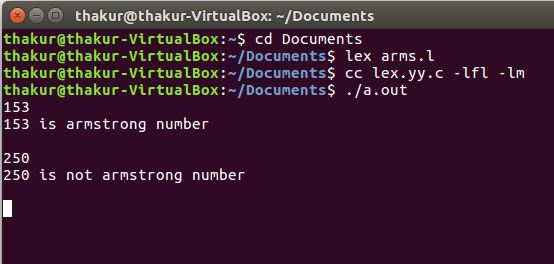# Lex program to check whether given number is armstrong number or not

Problem: Lex program to check whether given number is armstrong number or not.

Explanation:
Lex is a computer program that generates lexical analyzers and was written by Mike Lesk and Eric Schmidt. Lex reads an input stream specifying the lexical analyzer and outputs source code implementing the lexer in the C programming language.

Description:
An Armstrong number is a number that is the sum of its own digits each raised to the power of the number of digits. For example, 153 is an Armstrong number,

`(1^3) + (5^3) + (3^3) = 153 `

Examples:

```Input: 153
Output: 153 is a Armstrong number

Input: 250
Output: 250 is not a Armstrong number ```

Implementation:

 `/* Lex program to check whether given  ` `    ``- number is armstrong number or not */` `% ` `{ ` `/* Definition section */` `#include ` `#include ` `    ``void` `check(``char``*); ` `    ``% ` `} ` ` `  `/* Rule Section */` `% % ` `        ``[0 - 9] ` `    ``+ check(yytext); ` `% %  ` ` `  `int` `main() ` `{ ` `    ``/* yyin as pointer of File type */` `    ``extern` `FILE``* yyin; ` `    ``yyin = ``fopen``(``"num"``, ``"r"``); ` ` `  `    ``// The function that starts the analysis ` `    ``yylex(); ` ` `  `    ``return` `0; ` `} ` `void` `check(``char``* a) ` `{ ` `    ``int` `len = ``strlen``(a), i, num = 0; ` `    ``for` `(i = 0; i < len; i++) ` `        ``num = num * 10 + (a[i] - ``'0'``); ` ` `  `    ``int` `x = 0, y = 0, temp = num; ` `    ``while` `(num > 0) { ` `        ``y = ``pow``((num % 10), len); ` `        ``x = x + y; ` `        ``num = num / 10; ` `    ``} ` ` `  `    ``if` `(x == temp) ` `        ``printf``(``"%d is armstrong number \n"``, temp); ` `    ``else` `        ``printf``(``"%d is not armstrong number\n"``, temp); ` `} `

Output:Whether you're preparing for your first job interview or aiming to upskill in this ever-evolving tech landscape, GeeksforGeeks Courses are your key to success. We provide top-quality content at affordable prices, all geared towards accelerating your growth in a time-bound manner. Join the millions we've already empowered, and we're here to do the same for you. Don't miss out - check it out now!

Previous
Next